# Altova UModel 2023 Basic Edition

## Generate Code from Sequence Diagram

UModel can create code from a sequence diagram which is linked to at least one operation. Code generation from sequence diagrams is available for:

VB.NET, C# and Java

UModel standalone, Eclipse, and Visual Studio editions

All three UModel editions

Creating code from Sequence diagrams is possible by either:

Starting from a reverse engineered operation, see Generating Sequence Diagrams from source code,

By creating a new sequence diagram from scratch, which is linked to an operation, by right-clicking the operation (in the Model Tree) and selecting Create sequence diagram for code.

 When using a reverse engineered sequence diagram as basis, ensure that the option "Show code in notes" is selected when reverse engineering the code, so you do not lose any code when you start the forward-engineering process again. This is due to the fact that UML is not able to display all the language features of VB.NET, Java and C# on the sequence diagram, and those code sections are therefore shown as code notes.

To add plain text as code when creating a sequence diagram:

1.Attach a note to a sequence diagram lifeline.

2.Type in the code which should be written into the final source code. Click the Is Code check box (in the Properties pane) for that note, to make it accessible.

See Adding code to sequence diagrams for an example.

If a Sequence Diagram is to be used for code engineering automatically every time code engineering is started:

1.Select the diagram in the Model Tree or Diagram Tree window.

2.Select the Use for forward engineering check box in the Properties window.

Old code will always be lost when forward engineering code from a sequence diagram, because it will be overwritten with the new code.

To generate code using the Project menu:

1.Select the menu option Project | Generate Code from Sequence Diagrams. You are now prompted to select the specific Sequence Diagram(s). Clicking the "Select All" button selects all the Sequence Diagrams in the UModel project.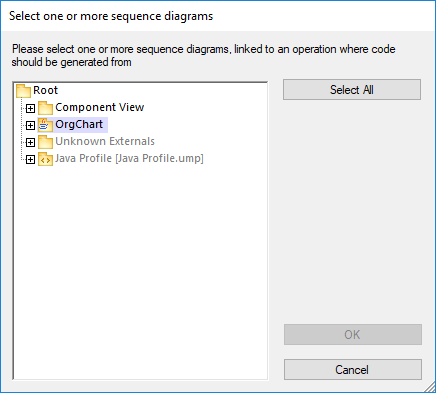2.Click OK to generate the code. The Messages window shows the status of the code generation process.

To generate code using the Model Tree:

Right click a Sequence Diagram and select Generate Code from Sequence diagram.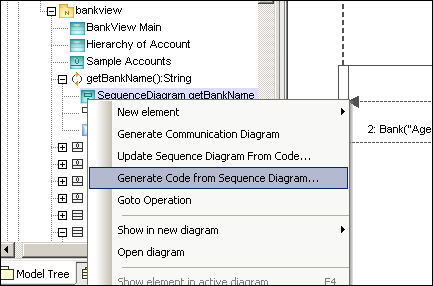To generate a Sequence Diagram containing code of an operation:

1.Click into the empty space of the Sequence Diagram, that contains code of an operation.

2.Select Generate Code from Sequence diagram.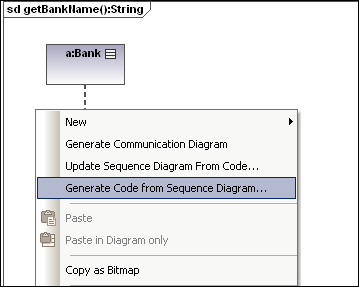This command starts the forward-engineering process at this point.

To create a Sequence diagram for code (engineering):

In the Model Tree, right-click an operation and select Create Sequence diagram for code.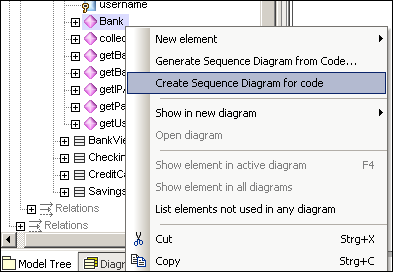You will then be prompted if you want to use the new diagram for forward engineering.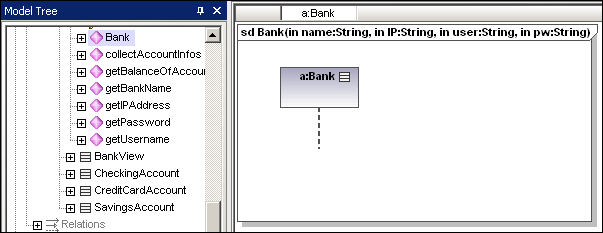The result is a new Sequence Diagram containing the lifeline of that class.